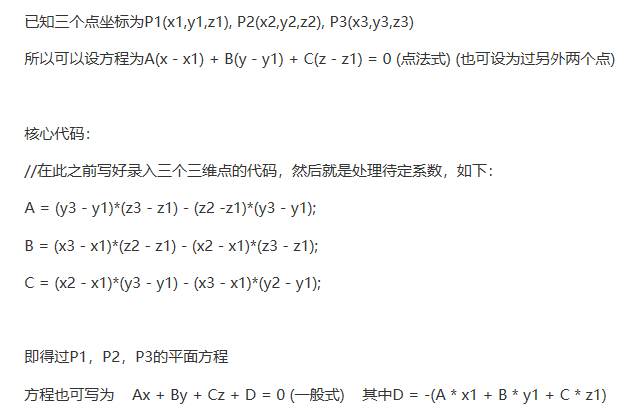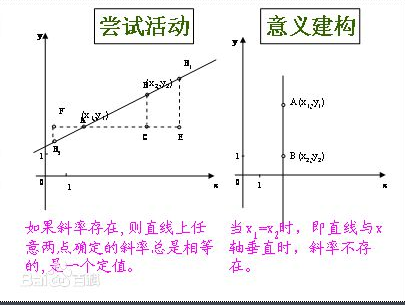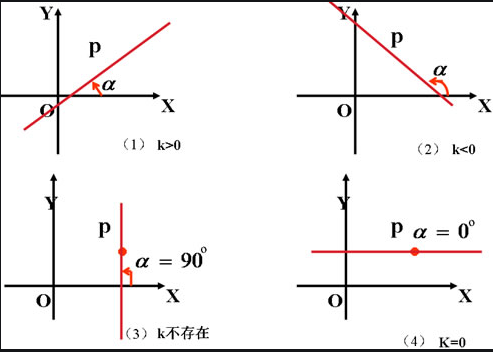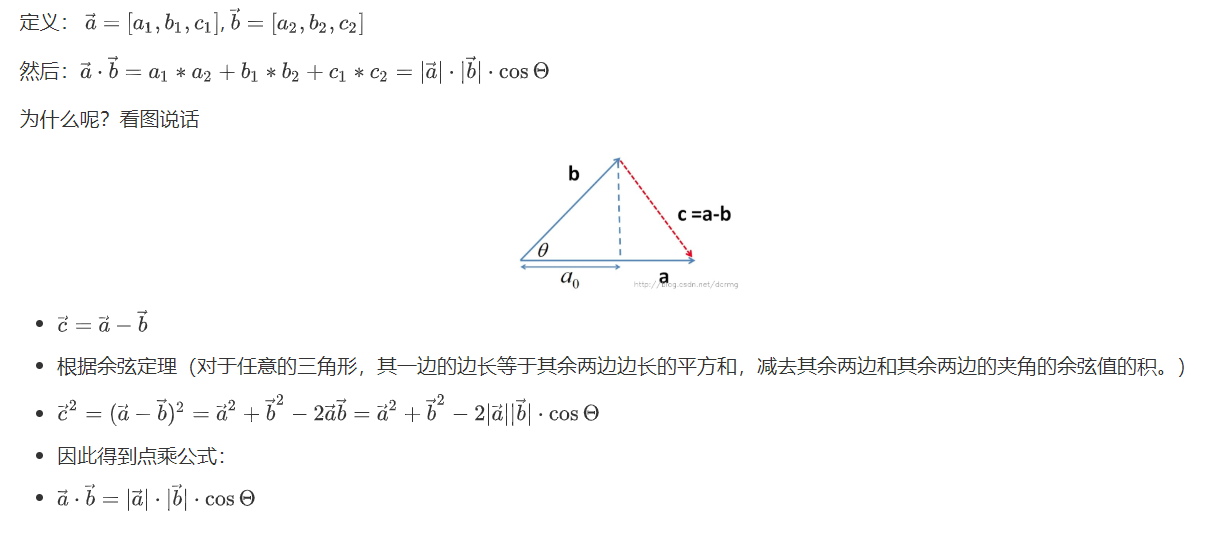• 创建直线 两式 一般式 点斜式 创建平面
程序设计小学期还要复习高数+线代，刺激
题目(2020/07/07)

第

8

天

：

空

间

点

、

线

和

面

第8天：空间点、线和面

实现三维解析几何中的点、直线和平面类
能够实现直线的不同创建方式（例如，两个点确定一条直线，两个相交的平面确定一条直线，空间曲线的点斜式）和平面的不同创建方式（例如，三个不共线的点确定一个平面，一个点和一个法向量确定一个平面）；能够计算相应的距离：两点之间的距离，点到直线的距离，点到平面的距离；能够计算空间直线的单位方向向量（长度为1），空间平面的单位法向量 （长度为1）；能够判断点和线的关系，线和线的关系，点和平面的关系，线和平面的关 系，平面和平面的关系。
提示：

1.点、直线和平面分别用类来封装，每个类的数据为该表示该类需要的参 数，例如，对于空间平面的一般方程为ax+by+cz+d=0，那么私有数据成 员为a,b,c和d； 2.空间直线和平面的不同创建方式定义为对应类的成员函数； 3.空间点和点、点和直线、点和平面、直线和直线、直线和平面、平面和平面位置关系的判断定义为一般的函数； 4.要在main函数中对上述功能进行调用和验证。

直线方面
两点式:
设两点为

A

(

x

1

,

x

2

,

x

3

)

A\left( x_{1},x_{2},x_{3}\right)

和

B

(

x

1

,

x

2

,

x

3

)

B\left(x_{1},x_{2},x_{3}\right)

，那么有方程为

x

−

x

1

x

2

−

x

1

=

y

−

y

1

y

2

−

y

1

=

z

−

z

1

z

2

−

z

1

\dfrac {x-x_{1}}{x_{2}-x_{1}}=\dfrac {y-y_{1}}{y_{2}-y_{1}}=\dfrac {z-z_{1}}{z_{2}-z_{1}}

一般式:

{

Π

1

:

A

1

x

+

B

1

y

+

C

1

z

+

D

1

=

0

Π

2

:

A

2

X

+

B

2

Y

+

C

2

z

+

D

2

=

0

\begin{cases}\Pi _{1}:A_{1}x+B_{1}y+C_{1}z+D_{1}=0\\ \Pi _{2}:A_{2}X+B_{2}Y+C_{2}z+D_{2}=0\end{cases}

点斜式:
方向向量：

S

→

=

(

m

,

n

,

p

)

\overrightarrow {S}=\left( m,n,p\right)

固定点：

M

0

(

x

0

,

y

0

,

z

0

)

M_{0}\left( x_{0},y_{0},z_{0}\right)

有：

M

0

M

→

=

(

x

−

x

0

,

y

−

y

0

,

z

−

z

0

)

\overrightarrow {M_{0}M}=\left( x-x_{0},y-y_{0},z-z_{0}\right)

x

−

x

0

m

=

y

−

y

0

n

=

z

−

z

0

p

\dfrac {x-x_{0}}{m}=\dfrac {y-y_{0}}{n}=\dfrac {z-z_{0}}{p}

平面方面
标准式：
固定点：

M

0

(

x

0

,

y

0

,

z

0

)

M_{0}\left( x_{0},y_{0},z_{0}\right)

A

(

x

−

x

0

)

+

B

(

y

−

y

0

)

+

C

(

z

−

z

0

)

=

0

A\left( x-x_{0}\right) +B\left( y-y_{0}\right) +C\left( z-z_{0}\right)=0

A

x

+

B

y

+

C

z

−

A

x

0

−

B

y

0

−

C

z

0

=

0

Ax+By+Cz-Ax_{0}-By_{0}-Cz_{0}=0

两点间距离公式 (送分 )

d

=

(

x

2

−

x

1

)

2

+

(

y

2

−

y

1

)

2

+

(

z

2

−

z

1

)

2

d=\sqrt {\left( x_{2}-x_{1}\right) ^{2}+\left( y_{2}-y_{1}\right) ^{2}+\left( z_{2}-z_{1}\right) ^{2}}

求点到线距离： （仅知道线上两点）
海伦公式：

S

=

p

(

p

−

a

)

(

p

−

b

)

(

p

−

c

)

S=\sqrt {p\left( p-a\right) \left( p-b\right) \left( p-c\right) }

公式描述：
公式中a，b，c分别为三角形三边长，p为半周长，S为三角形的面积。
点到面距离：

d

=

∣

A

x

0

+

B

y

0

+

C

z

0

+

D

∣

A

2

+

B

2

+

C

2

d=\dfrac {\left| Ax_{0}+By_{0}+Cz_{0}+D\right| }{\sqrt {A^{2}+B^{2}+C^{2}}}

空间直线的单位方向向量：

e

=

A

B

→

∣

A

B

→

∣

e=\dfrac {\overrightarrow {AB}}{\left| \overrightarrow {AB}\right| }

空间平面的单位法向量:

(

a

t

,

b

t

c

,

t

)

(

t

=

a

2

+

b

2

+

c

2

)

\left( \dfrac {a}{t},\dfrac {b}{t}\dfrac {c}{,t}\right) \left( t= \sqrt {a^{2}+b^{2}+c^{2}}\right)

已知三个点求平面方程：ps. 此图片来源于C++里已知三个三维点，求他们的平面方程，怎么做？
判断一些关系
点线位置关系：
海伦公式：

S

=

p

(

p

−

a

)

(

p

−

b

)

(

p

−

c

)

S=\sqrt {p\left( p-a\right) \left( p-b\right) \left( p-c\right) }

公式描述：
公式中a，b，c分别为三角形三边长，p为半周长，S为三角形的面积。
线线位置关系：

e

x

1

e

x

2

=

e

y

1

e

y

2

=

e

z

1

e

z

2

=

1

(

线

线

重

合

)

\dfrac {e_{x1}}{e_{x2}}=\dfrac {e_{y1}}{e_{y2}}=\dfrac {e_{z1}}{e_{z2}}=1\left( 线线重合\right)

e

x

1

e

x

2

=

e

y

1

e

y

2

=

e

z

1

e

z

2

≠

1

(

线

线

平

行

)

\dfrac {e_{x1}}{e_{x2}}=\dfrac {e_{y1}}{e_{y2}}=\dfrac {e_{z1}}{e_{z2}}\neq 1\left( 线线平行\right)

其

他

：

相

交

且

不

平

行

其他：相交且不平行

点面位置关系：
点到面距离：

d

=

∣

A

x

0

+

B

y

0

+

C

z

0

+

D

∣

A

2

+

B

2

+

C

2

d=\dfrac {\left| Ax_{0}+By_{0}+Cz_{0}+D\right| }{\sqrt {A^{2}+B^{2}+C^{2}}}

线面位置关系：

e

1

→

⋅

n

1

→

+

e

2

→

⋅

n

2

→

+

e

3

→

⋅

n

3

→

=

0

(

线

面

平

行

)

\overrightarrow {e_{1}}\cdot \overrightarrow {n_{1}}+\overrightarrow {e_{2}}\cdot \overrightarrow {n_{2}}+\overrightarrow {e_{3}}\cdot \overrightarrow {n_{3}}=0\left( 线面平行\right)

e

1

→

n

1

→

=

e

2

→

n

2

→

=

e

3

→

n

3

→

=

0

(

线

面

垂

直

)

\dfrac {\overrightarrow {e_{1}}}{\overrightarrow {n_{1}}}=\dfrac {\overrightarrow {e_{2}}}{\overrightarrow {n_{2}}}=\dfrac {\overrightarrow {e_{3}}}{\overrightarrow {n_{3}}}=0\left( 线面垂直\right)

面面位置关系：

n

x

1

n

x

2

=

n

y

1

n

y

2

=

n

z

1

n

z

2

≠

0

(

面

面

平

行

)

\dfrac {n_{x_{1}}}{n_{x_{2}}}=\dfrac {n_{y_{1}}}{n_{y_{2}}}=\dfrac {n_{z_{1}}}{n_{z_{2}}}\neq 0\left( 面面平行\right)

{

n

x

1

=

n

x

2

n

y

1

=

n

y

2

n

z

1

=

n

z

2

(

面

面

垂

直

)

\begin{aligned}\begin{cases}n_{x_{1}}=n_{x_{2}}\\ n_{y_{1}}=n_{y_{2}}\\ n_{z_{1}}=n_{z_{2}}\end{cases}\\ \left( 面面垂直\right) \end{aligned}

给出点线面的类定义：
class point
{
public:
double x,y,z;
void input(){cin>>x>>y>>z;};
void output(){cout<<"该点为："<<"("<<x<<","<<y<<","<<z<<")"<<endl;};
void dist();//求两点之间的距离
};
class line:public point//继承
{
public:
int m,n,p;//点斜式方向向量分量
int x,y,z;//点斜式点的坐标分量
point i,j;//每一条线由两个点确定
void Two_point_formula();//两点式
void Intersection_plane();
void P_oblique_type();
void D_point_to_line();
void L_vector();
};
class plane
{
public:
double a,b,c,d;
void setm();//赋值
void points_form_plane();//三点成面
void dot_method();//点法式
void showm();//方程式
void D_point_to_plane();//点到平面的距离
void n();//单位法向量
};

求两点之间的距离函数：
void point::dist()//求两点之间的距离
{
double l;
point m,n;
cout<<"请输入第一个点的坐标(x1,y1,z1): "<<endl;
m.input(); m.output();
cout<<"请输入第二个点的坐标(x2,y2,z2): "<<endl;
n.input(); n.output();
l=sqrt((n.x-m.x)*(n.x-m.x)+(n.y-m.y)*(n.y-m.y)+(n.z-m.z)*(n.z-m.z));
cout<<"两点之间的距离为= "<<l;
}

点到线(由两个点来表示直线)的距离
void line::D_point_to_line()//点到线(由两个点来表示直线)的距离
{//海伦公式
point y;
point d1,d2;
y.input();y.output();
d1.input();d1.output();
d2.input();d2.output();
double i1,i2,i3;
double p,s,l;
i1=sqrt((d1.x-d2.x)*(d1.x-d2.x)+(d1.y-d2.y)*(d1.y-d2.y)+(d1.z-d2.z)*(d1.z-d2.z));
i2=sqrt((d1.x-y.x)*(d1.x-y.x)+(d1.x-y.x)*(d1.y-y.y)+(d1.z-y.z)*(d1.z-y.z));
i3=sqrt((d2.x-y.x)*(d2.x-y.x)+(d2.y-y.y)*(d2.y-y.y)+(d2.z-y.z)*(d2.z-y.z));
p=(i1+i2+i3)/2;
s=sqrt(p*(p-i1)*(p-i2)*(p-i3));
l=2*s/i1;
cout<<l<<endl;
}

其他靠自己了😅
英语学习：

平面：plane 输入:input,输出:output,距离：dist(distance)
点到线的距离:D_point_to_line(Distancefrom point to line)
点到平面的距离:D_point_to_plane(Distancefrom point to plane)
直线单位方向向量:L_vector(Linearunit direction vector)
点线位置关系：P_line_position(Point line position relationship)
线线位置关系：Line_position(Line position relation)

点面位置关系：point_surface_Position(Positionrelation of point and surface)
线面位置关系：line_surface_Position(Positionrelation of line and surface)
面面位置关系：Surface_position(Surface position relation)

点斜式:P_oblique_type(Pointoblique type)
点法式:dot_method(dotmethod)
三点成面:points_form_plane(Threepoints form a plane)
两点式:Two_point_formula(Twopoint formula)
相交平面:Intersection_plane(Intersectionplane)

展开全文• 这里展示的是使用向量和三角函数来计算的线和面的交点 个人感觉代码里面使用向量和三角函数来计算比较方便写 /// <summary> /// 计算射线和面的交点 /// 会有一定误差 ， 浮点数计算没有办法 /// </...
关于射线和面相交的理论在这里无需赘述 这里展示的是使用向量和三角函数来计算的线和面的交点 个人感觉代码里面使用向量和三角函数来计算比较方便写
		/// <summary>
/// 计算射线和面的交点
/// 会有一定误差 ， 浮点数计算没有办法
/// </summary>
/// <param name="ray">射线</param>
/// <param name="normal">面的法线</param>
/// <param name="Point">面上的一点</param>
/// <param name="ret">交点</param>
/// <returns>线和面是否相交</returns>
bool intersectionOfRayAndFace(Ray ray, Vector3 normal,Vector3 Point, out Vector3 ret)
{
if (Vector3.Dot(ray.direction, normal) == 0)
{
//如果平面法线和射线垂直 则不会相交
ret = Vector3.zero;
return false;
}
Vector3 Forward = normal;
Vector3 Offset = Point - ray.origin; //获取线的方向
float DistanceZ = Vector3.Angle(Forward, Offset); //计算夹角
DistanceZ = Mathf.Cos(DistanceZ / 180f * Mathf.PI) * Offset.magnitude; //算点到面的距离
DistanceZ /= Mathf.Cos(Vector3.Angle(ray.direction, Forward) / 180f * Mathf.PI); //算点沿射线到面的距离
ret = ray.origin + ray.direction * DistanceZ; //算得射线和面的交点
return true;
}

展开全文unity3d c#
• 需要线、面生成缓冲区的又不要附加库依赖库，线、面处在不同的代码文件中，方便移植，不需要依赖库又要移植线、面缓冲区生成的最好代码；可直接运行~！
• 1.建立三个类，线、面。 2.在线和面的输入时，不同方式进行建立完成时，进行标准式进行标准化 3 运用数学知识进行进行线面关系，线线关系等关系的计算 4运用数学知识进行距离的计算。
• 假设有直线段L,直线上2分别为A(x1,y1)、B(x2,y2),那么直线段L的斜率k的取值范围是 ： -1 < k <1, k=(y2-y1) / (x2-x1); 如果有一点P(x,y)，判断p是否在直线L上，如果p在直接L上，那么： (y-y1）/ （x-x1）=k...
1、直线的斜率直线斜率(k)是直线与X轴右侧（正半轴方向）所成角的正切值。
  k=Math.tan(α)  =>       -无穷  < k < 无穷假设有直线段L,直线上已知2点分别为A(x1,y1)、B(x2,y2),那么直线段L的斜率：
k=tanα=（y2-y1）/（x2-x1）=（y1-y2）/（x1-x2）

如果有一点P(x,y)，判断P是否在直线L上， ①如果P在直接L上，那么P点和A点以及P和B点构成的线段斜率都和直线L的斜率相等
   (y-y1）/ （x-x1）= k  =y2-y1）/（x2-x1）=（y1-y2）/（x1-x2） =>
(y-y1) / (x-x1) - (y2-y1) / (x2-x1)=0

②、如果点P不在直线上，如何判断点P在直线的哪一边？ (y-y1) / (x-x1) -(y2-y1) / (x2-x1) > 0 ,点P在线段的左边 (y-y1) / (x-x1) -(y2-y1) / (x2-x1) < 0, 点P在线段的右边
以上换成代码： 1、如果直线的斜率不存在,A和B两点的X坐标相等：
 // 直线斜率k=tanα=（y2-y1）/（x2-x1）=（y1-y2）/（x1-x2）
/**
* 判断点和直线的关系
* 已知直线L上2点 A(x1,y1)、B(x2,y2),求点P(x,y)和直线的关系：①、点在直线上; ②、点在直线左边; ③、点在直线右边
* @param {*} A 已知坐标的直线上一点A
* @param {*} B 已知坐标的直线上一点B
* @param {*} P P是需要判断和直线关系的点,坐标已知
*/
function pointWithLineRelation(P,A,B){
let x1=A.x,y1=A.y,
x2=B.x,y2=B.y,
x=P.x,y=P.y ;
if(x1!==x2){
//直线斜率存在
if(y1===y1){
//斜率为0
if(y===y1){
console.log('点在直线上');
return 'onLine';
}else if(y < y1){
console.log('点在直线下面');
return 'bottom';
}else{
console.log('点在直线上面');
return 'top';
}
}
let k=(y2-y1) / (x2-x1);//直线斜率 k=(y2-y1) /(x2-x1)=(y1-y2) /(x1-x2)
//求P和直线上任一点的斜率 kx
let kx=(y-y1) / (x-x1);
if(kx - k===0){
console.log('点在直线上');
return 'onLine';
}else if(kx - k >0){
console.log('点在直线左侧');
return 'left';
}else if(kx - k <0){
console.log('点在直线右侧');
return 'right';
}
}else{
//直线斜率不存在 x1===x2
if(x===x1){
console.log('点在直线上');
return 'onLine';
}else if(x < x1){
console.log('点在直线左侧');
return 'left';
}else{
console.log('点在直线右侧');
return 'right';
}

}

}
/**
* 判断2条线段是否相交，假设有线段L1,顶点分别为A1、B1;线段L2,顶点分别为A2、B2。
* 数学定理：如果线段L1和L2相交，那么线段L1的2个顶点应该分别位于线段L2的两侧，并且线段L2的2个顶点也应该分别位于线段L1的2侧，
*           否则，2条线段就是不相交的。
*
* @param {*}  coordArry1  线段L1的顶点坐标  形如[{x:2,y:0},{x:5,y:5}]
* @param {*}  coordArry2  线段L2的顶点坐标  形如[{x:4,y:0},{x:7,y:8}]
*/
function linWithLineRelation(coordArry1,coordArry2){
let L1p1=coordArry1,L1p2=coordArry1; //线段L1上的2个顶点L1P1、L1P2
let L2p1=coordArry2,L2p2=coordArry2; //线段L1上的2个顶点L1P1、L1P2
let k1=(L1p2.y - L1p1.y) / (L1p2.x - L1p1.x);
let k2=(L2p2.y - L2p1.y) / (L2p2.x - L2p1.x);
if(k1===k2){
console.log('2条线段斜率相等,不相交');
return false;
}
//求线段L1的第1个顶点和线段L2的关系
let L1p1WithL2=pointWithLineRelation(L1p1,L2p1,L2p2);
//求线段L1的第2个顶点和线段L2的关系
let L1p2WithL2=pointWithLineRelation(L1p2,L2p1,L2p2);

//求线段L2的第1个顶点和线段L1的关系
let L2p1WithL1=pointWithLineRelation(L2p1,L1p1,L1p2);
//求线段L2的第2个顶点和线段L1的关系
let L2p2WithL1=pointWithLineRelation(L2p2,L1p1,L1p2);
if(L1p1WithL2 !==L1p2WithL2 && L2p1WithL1!==L2p2WithL1){
console.log('2条线段不相交');
return false;
}else{
console.log('2条线段相交');
return true;
}
}

/**
* 求空间面polygon1 和面polygon2 是否相交，可以把面和面的相交分解为求线段和线段是否相交,分别把 polygon1按每2个相邻顶点拆分成线，polygon2也同样按每2个相邻顶点拆分成线；然后用 polygon1拆分后的线段逐一 和 polygon2拆分后的每一条线段做相交关系判断,如果polygon1每一条线段都和polygon2的其中任何一条线段没有交点，那么2个面就是不相交的，否则，2个面就是相交的。
*
*/


展开全文• 和面的交线 **1.*面的方程一般式为：Ax+By+Cz+D=0 (参数,A,B,C,D是描述平面空间特征的常数)， 其中（A,B,C)为平面的法向量，D为将已知带入得到的补偿值。 2.1求取面与面的交线，已知条件：法向量和已知 2.2...
1 面的定义 三维空间中的平面由两个量确定： ① 一个法向量（垂直于该平面的向量） ② 一个已知点（位于该平面上的一个点 2 叉乘和点乘的区别
2.1叉乘的计算方式，叉乘用来得到垂直于两条向量的向量。2.2点乘的计算方式，内积（点乘）的几何意义包括：表征或计算两个向量之间的夹角，b向量在a向量方向上的投影.面和面的交线 **1.*面的方程一般式为：Ax+By+Cz+D=0 (参数,A,B,C,D是描述平面空间特征的常数)， 其中（A,B,C)为平面的法向量，D为将已知点带入得到的补偿值。 2.1求取面与面的交线，已知条件：法向量和已知点 2.2公式推导： 平面1：a1x+b1y+c1z+d1=0；平面2：a2x+b2y+c2z+d2=0 平面法向量;n1=(a1,b1,c1),n2=(a2,b2,c2) 交线的方向向量n=n1×n2=(b1c2-c1b2,c1a2-a1c2,a1b2-b1a2) 设直线上任意一点为（x,y,z） 令x=0,得： b1y+c1z+d1=0，b2y+c2z+d2=0, 即 y=-(c1z+d1)/b1=-(c2z+d2)/b2 解得： z=-(d1/b1-d2/b2)/(c1/b1-c2/b2) y=-c1/b1z-d1/b1 =c1(d1/b1-d2/b2)/b1/(c1/b1-c2/b2)-d1/b1 由直线方向向量和一点坐标即可确定该直线。 3 python代码如下
       #两个面 Axial 和 Coronal ，两个面的法向量为normalAxial和normalCoronal，两个面上的点为positionAxial和positionCoronal
#对两个面的法向量进行叉乘，得到交线的方向向量
vectorAC = np.cross(normalAxial, normalCoronal)
print(vectorAC)
# axial plane
a1=normalAxial
b1=normalAxial
c1=normalAxial
d1 = -np.dot(positionAxial,normalAxial)
# coronal plane
a2=normalCoronal
b2=normalCoronal
c2=normalCoronal
d2 = -np.dot(positionCoronal,normalCoronal)
if  normalCoronal!=0 and normalCoronal!=0:
print("平面旋转计算中")
# tempz = -(d1 / b1 - d2 / b2) / (a1 / b1 - a2 / b2)
tempz = -d1
print("tempz",tempz)
# tempz = (-a1 / b1) * tempz - d1 / b1
tempy = -(d2)/b2
print("tempy,",tempy)
centerPointACOne= [0,tempy,tempz]
print("平面旋转计算中01,centerPointACOne",centerPointACOne)
#由此得到交线的一点，再加上交线的方向向量，可以得到平面的交线方程了。

面和线的交点 我们假设它们的交点为P， 既然我们有一个平面，那么平面上面的一个点planePoint和平面的planeNormal（垂直于平面的向量）已知。根据3D数学知识，

（

P

−

p

l

a

n

e

P

o

i

n

t

)

⋅

p

l

a

n

e

N

o

r

m

a

l

=

0

（

公

式

一

）

（P-planePoint) · planeNormal= 0（公式一）

既然垂直，那么它们点乘肯定为 对于这条直线，我们肯定知道直线上面的某一点linePoint和直线的方向lineVector，那么

P

=

l

i

n

e

P

o

i

n

t

+

d

∗

l

i

n

e

V

e

c

t

o

r

（

公

式

二

）

P = linePoint+ d*lineVector（公式二）

d是距离。把公式二代入公式一， 我们可以得到如下：

（

l

i

n

e

P

o

i

n

t

+

d

∗

l

i

n

e

V

e

c

t

o

r

−

p

l

a

n

e

P

o

i

n

t

）

∗

p

l

a

n

e

N

o

r

m

a

l

=

0

（linePoint+ d*lineVector-planePoint）*planeNormal= 0

由此得到

d

∗

l

i

n

e

V

e

c

t

o

r

∗

p

l

a

n

e

N

o

r

m

a

l

+

(

l

i

n

e

P

o

i

n

t

−

p

l

a

n

e

P

o

i

n

t

)

∗

p

l

a

n

e

N

o

r

m

a

l

=

0

d*lineVector*planeNormal+ (linePoint- planePoint)*planeNormal= 0

这样我们可以求出d值，然后我们就可以通过公式二求出P啦！
展开全文线性代数
• 为AAA、BBB，齐次坐标系为 A=[x1,y1,z1,1]TA=[x_1,y_1,z_1,1]^TA=[x1​,y1​,z1​,1]T、B=[x2,y2,z2,1]TB=[x_2,y_2,z_2,1]^TB=[x2​,y2​,z2​,1]T。 平面为π\piπ，齐次表示为π=[π1,π2,π3,π4]T\pi=[\pi_1...线性代数
• 空间中面的距离 有些时候我们在看计算机一些书籍的时候经常会涉及到空间中点与面之间的关系，书中会突然出现一些公式，可能很多时候我们会忘了证明的方法。 今天来谈谈空间中面的公式。 下面先给出空间...
• 各位大婶 本人GIS专业大三学生一枚 实习中遇到任务 要基于MO的开发环境实现线、面要素的空间查询？因为以前学的比较渣 然后现在对于VS操作步骤基本不会 然后对于代码也是一脸茫然 跪求会的大神在百忙之中能知道...代码 操作 设计
• 空间两直线交点-MATLAB代码+原理说明 原理.pdf —— 说明性文档 main_demo.m —— demo主程序，也就是一个示例 Intersection_of_TwoLines.m —— 求交点的函数MATLAB 几何
• ## 点线面拓扑关系

千次阅读 2018-08-31 18:53:21
主要有六大关系：点点关系、点线关系、面关系、线线关系、线面关系、面面关系 线 / 线 /线 线/线/面 线/面 面/面 ...拓扑关系 九交模型
•   在三维空间中，最基本的元素莫过于基本的线和面。   他们之间的关系，可以这样理解，按着一定的方向平移可以得到直线，线按照一定的方向平移可以得到平面，这个结论想象一下就可以理解了。 ​ 可是要...
• ---------------------  作者：傻子与倩倩  来源：CSDN  ...版权声明：本文为博主原创文章，转载请附上博文... 工业相机按照传感器的结构特性可分为面阵相机和线阵相机，面阵、线阵相机都有各自的优点和缺点，在用...面阵相机
• ## WPF开发教程

万次阅读 多人点赞 2019-07-02 23:13:20
5. XAML命名空间和命名空间映射... 90 6. WPF名称范围... 92 WPF控件开发.... 95 1. WPF控件开发之控件概述... 95 2. 使用XAML创建按钮... 103 3. WPF控件库之Button. 114 4. WPF控件库之...WPF
• 了解线阵相机与面阵相机的基本区别  工业相机按照传感器的结构特性可分为面阵相机和线阵相机，面阵、线阵相机都有各自的优点和缺点，在用途不同的情况下选择合适的传感器的结构工业相机，至关重要。 1、类型区分 面...机器视觉
• 这一篇作为线性代数栏目的扩展篇之一，是因为随着学习的深入，必须提前学习一些具有应用针对性的数学计算方法，这次我们就来观察立体几何空间中点和面的关系，主要还是为了CG中着色应用，比如真实反射和光追计算。...
• 目录第一节 空间数据查询第二节 缓冲区分析第三节 叠置分析第四节 网络分析第五节 DEM建立及分析 第一节 空间数据查询 1.1 空间数据查询 1、空间数据查询的含义 从空间数据库中找出所有满足属性约束条件和空间约束...ArcGIS 缓冲区分析 叠置分析 网络分析
• 数据挖掘中有些算法，特别是分类算法，只能在离散型数据上进行分析，然而大部分数据集常常是连续值和离散值并存的。...等频分箱法的思想是，将观察均匀分成n等份，每份的观察数相同。 在对数据离散化前，机器学习 python
• 可以表示平面上的任意一条直线缺点：要确定的常数较多斜截式y=kx+b优点：只需要斜率和截距缺点：不能表示垂直x轴的直线x=a点斜式y-y0=k(x-x0)优点：只需要一个和斜率缺点：不能表示垂直x轴的直线x=a两式(y-y1)/...
• 1. 空间点线和面： 实现三维解析几何中的、直线和平面类 (1) 能够实现直线的不同创建方式（例如，两个确定一条直线，两个相交的平面确定一条直线，空间曲线的点斜式）和平面的不同创建方式（例如，三个不共...c++ c语言
• 口诀： 最后是 “0”的结果 垂直 叉 平行 数量积：a·b = |a||b|cos(a,b)= a1b1+a2b2+a3b3，两个向量的数量积是一个数 两个向量平行充分必要条件：a //b <=>a = kb 或a //b <=>axb = 0; 叉乘 ...
• 　sdo_Geometry1，sdo_Geometry2为空间数据对应的几何对象。 　Tolerance: 容许的精度范围； --  Return the topological intersection of two geometries. SELECT  SDO_GEOM.SDO_INTERSECTION...oracle
• 在计算机世界里，3D世界是由组成，两个能够组成一条直线，三个不在一条直线上的就能够组成一个三角形面，无数三角 面可以构造任何3D物体 我们通常把这种网格模型叫做Mesh模型。给物体贴上皮肤，或者专业就...javascript 前端 WebGL
• 如下图所示，已知直线L过m（m1，m2，m3），且方向向量为VL（v1，v2，v3），平面P过n（n1，n2，n3），且法线方向向量为VP（vp1，vp2，vp3），求得直线与平面的交点O的坐标（x，y，z）： 将直线方程写成参数......